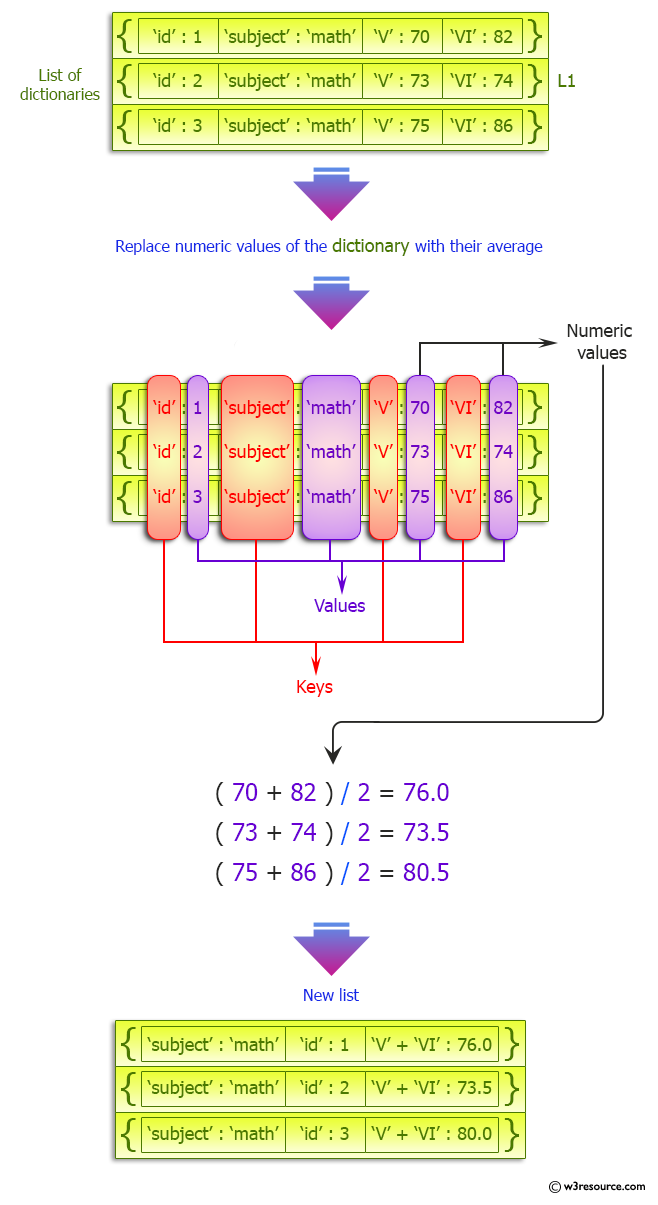﻿ Python: Replace dictionary values with their average - w3resource# Python: Replace dictionary values with their average

## Python dictionary: Exercise-37 with Solution

Write a Python program to replace dictionary values with their average.

Sample Solution:-

Python Code:

``````def sum_math_v_vi_average(list_of_dicts):
for d in list_of_dicts:
n1 = d.pop('V')
n2 = d.pop('VI')
d['V+VI'] = (n1 + n2)/2
return list_of_dicts
student_details= [
{'id' : 1, 'subject' : 'math', 'V' : 70, 'VI' : 82},
{'id' : 2, 'subject' : 'math', 'V' : 73, 'VI' : 74},
{'id' : 3, 'subject' : 'math', 'V' : 75, 'VI' : 86}
]
print(sum_math_v_vi_average(student_details))
```
```

Sample Output:

```[{'subject': 'math', 'id': 1, 'V+VI': 76.0}, {'subject': 'math', 'id': 2, 'V+VI': 73.5}, {'subject': 'math', '
id': 3, 'V+VI': 80.5}]
```

Pictorial Presentation:## Visualize Python code execution:

The following tool visualize what the computer is doing step-by-step as it executes the said program:

Python Code Editor:

Have another way to solve this solution? Contribute your code (and comments) through Disqus.

What is the difficulty level of this exercise?

Test your Programming skills with w3resource's quiz.

﻿

## Python: Tips of the Day

Invokes the provided function after ms milliseconds:

Example:

```from time import sleep

def tips_delay(fn, ms, *args):
sleep(ms / 1000)
return fn(*args)
print(tips_delay(
lambda x: print(x),
1000,
'w3r'
))
```

Output:

```w3r
None
```